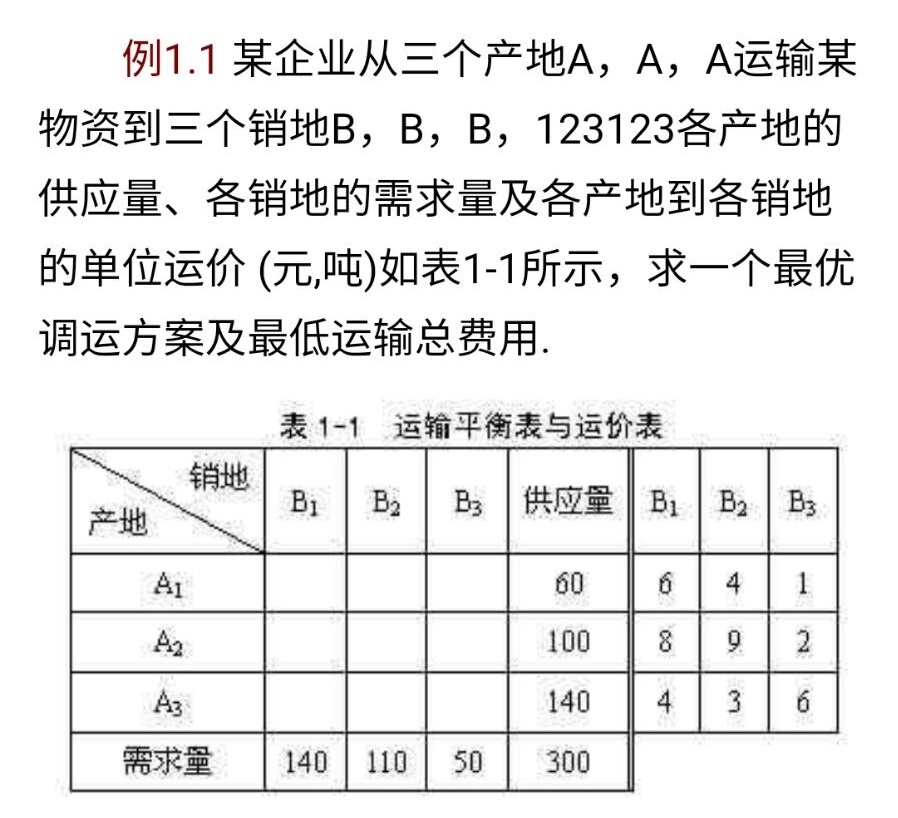2021-06-23 10:26

# 运筹学中的运输问题matlab• 点赞
• 写回答
• 关注问题
• 收藏
• 邀请回答

#### 1条回答默认 最新

•CSDN专家-Matlab_Fans 2021-06-23 12:48
已采纳

将上述问题转换为线性规划问题：

1. 设xij为Ai运输至Bj的货物量，设P=[6 4 1 8 9 2 4 3 6]为与x对应的单价，因此目标函数为最小化P'*x

2. 约束条件 sum(x1j) = 60，sum(x2j) = 100 …… sum(i3) = 50，共6个等式约束。

使用matlab求解上述线性规划问题

``````% 目标函数
f = [6 4 1 8 9 2 4 3 6]';

% 约束条件
m = 3;
n = 3;
Aeq1 =  repmat( eye(m),1,n );
beq1 = [140 110 50]';
Aeq2 =  kron( eye(m),[1 1 1] );
beq2 = [60 100 140]';
Aeq = [Aeq1;Aeq2];
beq = [beq1;beq2];
lb = zeros(m*n,1);       % 下限
ub = 200*ones(m*n,1);    % 上限

x = linprog(f,[],[],Aeq,beq,lb,ub)
y = f'*x
``````

结果：

``````
x =

0
60
0
50
0
50
90
50
0

y =

1250``````
点赞 评论